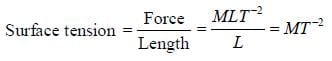The dimensional formula of surface tension in...

### Related TestThe dimensional formula of surface tension in terms of the dimensions of mass (M), length (L) and time (T) is
• a)
MT-2
• b)
MLT-2
• c)
MT-1
• d)
ML-1T-2SEEMA JAINView courses related to this question Explore IIT JAM courses
 Explore IIT JAM coursesView courses related to this question1 Crore+ students have signed up on EduRev. Have you?

• ### A cube X, with each side of 2 cm length, is split into two new objects Y an... more

Question Description
The dimensional formula of surface tension in terms of the dimensions of mass (M), length (L) and time (T) isa)MT-2b)MLT-2c)MT-1d)ML-1T-2Correct answer is option 'A'. Can you explain this answer? for IIT JAM 2023 is part of IIT JAM preparation. The Question and answers have been prepared according to the IIT JAM exam syllabus. Information about The dimensional formula of surface tension in terms of the dimensions of mass (M), length (L) and time (T) isa)MT-2b)MLT-2c)MT-1d)ML-1T-2Correct answer is option 'A'. Can you explain this answer? covers all topics & solutions for IIT JAM 2023 Exam. Find important definitions, questions, meanings, examples, exercises and tests below for The dimensional formula of surface tension in terms of the dimensions of mass (M), length (L) and time (T) isa)MT-2b)MLT-2c)MT-1d)ML-1T-2Correct answer is option 'A'. Can you explain this answer?.
Solutions for The dimensional formula of surface tension in terms of the dimensions of mass (M), length (L) and time (T) isa)MT-2b)MLT-2c)MT-1d)ML-1T-2Correct answer is option 'A'. Can you explain this answer? in English & in Hindi are available as part of our courses for IIT JAM. Download more important topics, notes, lectures and mock test series for IIT JAM Exam by signing up for free.
Here you can find the meaning of The dimensional formula of surface tension in terms of the dimensions of mass (M), length (L) and time (T) isa)MT-2b)MLT-2c)MT-1d)ML-1T-2Correct answer is option 'A'. Can you explain this answer? defined & explained in the simplest way possible. Besides giving the explanation of The dimensional formula of surface tension in terms of the dimensions of mass (M), length (L) and time (T) isa)MT-2b)MLT-2c)MT-1d)ML-1T-2Correct answer is option 'A'. Can you explain this answer?, a detailed solution for The dimensional formula of surface tension in terms of the dimensions of mass (M), length (L) and time (T) isa)MT-2b)MLT-2c)MT-1d)ML-1T-2Correct answer is option 'A'. Can you explain this answer? has been provided alongside types of The dimensional formula of surface tension in terms of the dimensions of mass (M), length (L) and time (T) isa)MT-2b)MLT-2c)MT-1d)ML-1T-2Correct answer is option 'A'. Can you explain this answer? theory, EduRev gives you an ample number of questions to practice The dimensional formula of surface tension in terms of the dimensions of mass (M), length (L) and time (T) isa)MT-2b)MLT-2c)MT-1d)ML-1T-2Correct answer is option 'A'. Can you explain this answer? tests, examples and also practice IIT JAM tests.(Scan QR code)